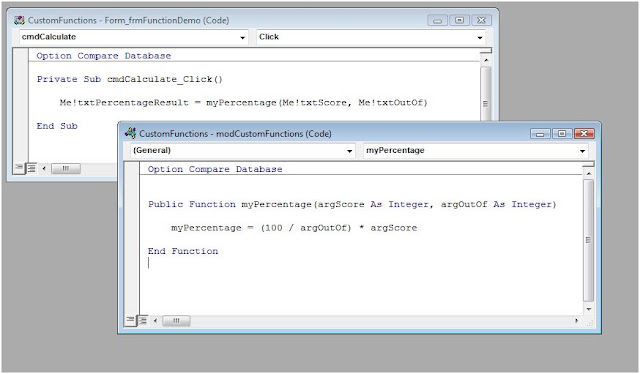## Friday, 25 May 2012

### Writing Custom Functions for Access VBA

VBA Custom Functions work in a similar way to any inbuilt MS Access Function.  Both types can be called within VBA sub-procedures1, and then return a value for the function's 'result'.  Take the inbuilt DLookUp function, for example.  This is written as follows:

varResult = DLookUp ( parameter1, parameter2, parameter3 )

Three things happen when we call this function:
1. The function is called using VBA code (passing three parameters in the process),
2. The function looks up information stored in an access table (based on the parameters passed),
3. It then returns that information, storing the result in a variable called varResult.
On account of DLookUp being a built in function, we do not get to see what happens in stage two.  Custom Functions are different in that we actually write a block of VBA code that executes at that stage.  Once we have set this up, we can call our custom function as often as we like.  Moreover, because custom functions tend to be located in a VBA global module, and declared as 'Public' in scope, they can be called from multiple points within our application. In this way, the creation of custom functions make our programming more organized and manageable.Figure 1:  This screenshot of the VBA Project Explorer shows  a module call modCustomFunction.  Providing the custom functions contained within are declared 'public' they can be called from anywhere within the project  - such as the form which I have called frmFunctionDemo.

How to Create a Custom Function
When we create a custom function we need to write the code that executes once it has been called from a sub procedure. As we shall see, this code is structured in a similar manner to an ordinary sub.  However, we also need to include a line of code which specifically returns a value back to the calling code. This is done by assigning a value to the name of the function (in a similar manner to assigning a value to a variable). For example:

Public Function myPercentage(argScore As Integer, argOutOf As Integer)
myPercentage = (100 / argOutOf) * argScore 2
End Function

This creates a function called myPercentage.  The first line of code declares the function, and receives two arguments from the calling code ie argScore and argOutof.  The second line of code in this example returns the result of the myPercentage function.  There can any number of lines of code preceding the returning line.  However, I have provided a simple example which returns the result of the function in the same line of code as a percentage calculation.  This code is stored in a global module called modCustomFunctions (see Figure 1 above).

Here is the code I have used to call this function:

Private Sub cmdCalculate_Click()
Me!txtPercentageResult = myPercentage(Me!txtScore, Me!txtOutOf)
End Sub

This code executes when a form command button (cmdCalculate) is clicked by the user.  The function (myPercentage) is called in the second line of code.  As you can see, the calling code for myPercentage passes two parameters, the values of which reference two text box controls - txtScore and txtOutOf.  The function uses these parameters (which are received as the arguments argScore and argOutOf) to calculate the percentage and return the result.  The result is then displayed in a third text box called txtPercentageResult.Figure 2: This is the form from which our function is called.  The CORRECT ANSWERS textbox is called txtScore, No OF QUESTIONS is called txtOutOf, and the PERCENTAGE textbox is called txtPercentageResult.  The CALCULATE button is called cmdCalculate.Figure 3: The top window shows the code calling the myPercentage function.  The lower window shows the code from the myPercentage function itself.

Footnote

1/ It needs to be pointed out here that the inbuilt functions of MS Access can be can be called from within Access itself (eg within the Query Design Grid as a criteria, or to populate a calculated control on a form etc), and not just from within VBA.  Custom functions, on the other hand, can only be called within VBA.